Note: This is an archvied version of our old webpage. Some links might be broken. The current one can be found here.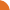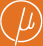Chair for Foundations of Software Reliability and Theoretical Computer Science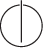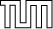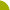Publications - Approximative Methods for Monotone Systems of min-max-Polynomial EquationsReference:

Javier Esparza, Thomas Gawlitza, Stefan Kiefer, and Helmut Seidl. Approximative methods for monotone systems of min-max-polynomial equations. In Luca Aceto et al., editor, Proceedings of the 35th International Colloquium on Automata, Languages and Programming (ICALP), part I, volume 5125 of LNCS, pages 698–710, Reykjavik, Iceland, 2008. Springer.

Abstract:

A monotone system of min-max-polynomial equations (min-max-MSPE) over the variables X_1, ..., X_n has for every i exactly one equation of the form X_i = f_i(X_1, ..., X_n) where each f_i(X_1, ..., X_n) is an expression built up from polynomials with non-negative coefficients, minimum- and maximum-operators. The question of computing least solutions of min-max-MSPEs arises naturally in the analysis of recursive stochastic games. Min-max-MSPEs generalize MSPEs for which convergence speed results of Newton's method have been established in previous papers. We present the first methods for approximatively computing least solutions of min-max-MSPEs which converge at least linearly. Whereas the first one converges faster, a single step of the second method is cheaper. Furthermore, we compute epsilon-optimal positional strategies for the player who wants to maximize the outcome in a recursive stochastic game.

Suggested BibTeX entry:

@inproceedings{EGKS08:icalp,
author = {Javier Esparza and Thomas Gawlitza and Stefan Kiefer and Helmut Seidl},
booktitle = {Proceedings of the 35th International Colloquium on Automata, Languages and Programming (ICALP), part I},
editor = {Luca Aceto et al.},
pages = {698--710},
publisher = {Springer},
series = {LNCS},
title = {Approximative Methods for Monotone Systems of min-max-Polynomial Equations},
volume = {5125},
year = {2008}
}GZipped PostScript (115 kB)PDF (147 kB)Tech report version